• 着两天只使用了一丢丢,就感叹到Vector的博大精深啊,奈何我这木鱼脑袋.查阅资料外加自己悟.对我用到的部分进行记录. 1.1实例化 简约版: vector<int>temp1; temp1.push_back(8); vec3d temp2;//实际是定义...

1.使用

着两天只使用了一丢丢,就感叹到Vector的博大精深啊,奈何我这木鱼脑袋.查阅资料外加自己悟.对我用到的部分进行记录.

1.1实例化

简约版:

vector<int>temp1;

temp1.push_back(8);

vec3d temp2;//实际是定义一个长度为3的数组

temp2=Vec3d(22,44,88);//初始化必须这样操作//访问每一个元素如下

cout<<temp2<<endl;//2

cout<<temp2<<endl;//2

cout<<temp2<<endl;//2

复杂版

vector<Vec2f>temp3;

temp3.push_back(Vec2f(3,4));

temp3.push_back(Vec2f(5,6));

cout<<temp3<<endl;

//可以通过temp[]来访问,不能用来赋值

升级版

vector<type>temp4;

temp4.push_back(type())

展开全文opencv vector
• 功能：vector容器的功能和数组非常相似，使用时可以把它看成一个数组 vector和普通数组的区别： 1.数组是静态的，长度不可改变，而vector可以动态扩展，增加长度 2.数组内数据通常存储在栈上，而vector中数

vector容器的基本概念

功能：vector容器的功能和数组非常相似，使用时可以把它看成一个数组

vector和普通数组的区别：
1.数组是静态的，长度不可改变，而vector可以动态扩展，增加长度
2.数组内数据通常存储在栈上，而vector中数据存储在堆上

动态扩展：(这个概念很重要)
动态扩展并不是在原空间之后续接新空间，而是找到比原来更大的内存空间，将原数据拷贝到新空间，释放原空间

注意：使用vector之前必须包含头文件 #include<vector>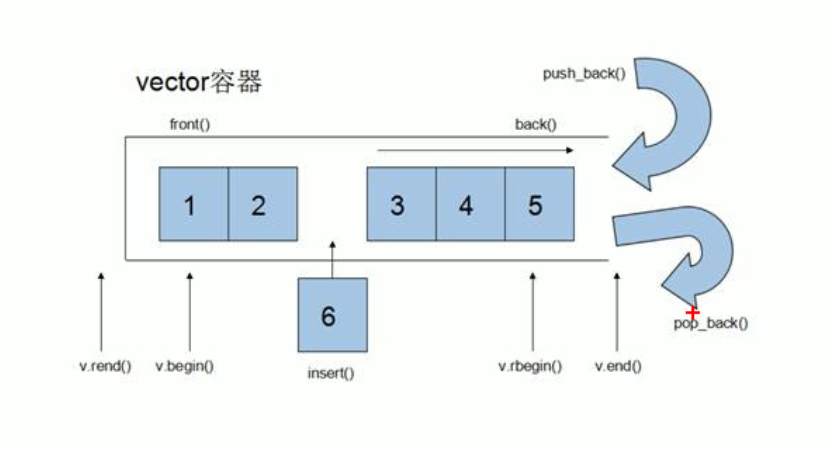1.vector的构造函数

函数原型：
1.vector<T> v ; //使用模板类，默认构造函数
2.vector(v.begin(),v.end()); //将[v.begin(),v.end())区间中的元素拷贝给本身
3.vextor(n,elem); //将n个elem拷贝给本身
4.vector(const vector &v) ; //拷贝构造函数

为了方便测试，我们先定义一个打印输出的函数，利用迭代器实现，这个函数下面会经常使用，迭代器可以理解为指针

void printVector(vector<int>& v)
{	//利用迭代器打印 v
for (vector<int>::iterator it = v.begin(); it != v.end(); ++it)
{
cout << *it << " ";
}
cout << endl;
}

测试案例：

void text01()
{
vector<int> v1;                       //调用1
for (int i = 0; i < 5; ++i)
{
v1.push_back(i);//向v1末尾添加数据
}
vector<int> v2(v1.begin(), v1.end());//调用2
vector<int> v3(5, 5);                //调用3
vector<int> v4(v3);                  //调用4
cout << "打印v2: ";
printVector(v2);
cout << "打印v3: ";
printVector(v3);
cout << "打印v4: ";
printVector(v4);
}

测试结果：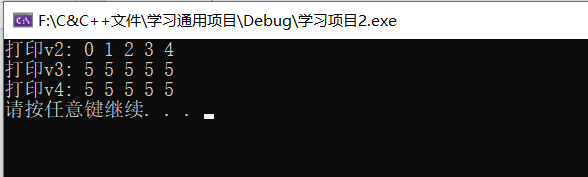2.vector的赋值操作

函数原型：
1.vector& operator=(const vector &v); //重载赋值运算符
2.assign(v.begin(),v.end()); //将[v.begin(),v.end())区间中的元素赋值给本身
3.assign(n,elem); //将n个elem赋值给本身

测试案例：

void text02()
{
vector<int> v1,v2;
for (int i = 0; i < 5; ++i)
{
v1.push_back(i);
}
v2 = v1;                        //调用1，赋值运算符重载
vector<int> v3,v4;
v3.assign(v1.begin(), v1.end());//调用2，区间赋值
v4.assign(5, 9);                //调用3
cout << "打印v2: ";
printVector(v2);
cout << "打印v3: ";
printVector(v3);
cout << "打印v4: ";
printVector(v4);
}

测试结果：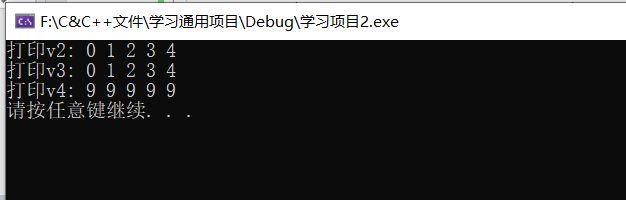3.vector的容量与大小

函数原型：
1.empty(); //判断容器是否为空，为空返回1，否则返回0
2.capacity(); //返回容器的容量
3.size(); //返回容器的大小,即容器中元素的个数
4.resize(int num); //重新指定容器的长度为num，若容器变长，则以默认值0填充新位置,如果容器变短，则末尾超过容器长度的元素被删除
5.resize(int num,int elem); //重新指定容器的长度为num，若容器变长，则以elem填充新位置,如果容器变短，则末尾超过容器长度的元素被删除
resize特性:长赋值，短截断

测试案例：

void text03()
{
vector<int> v1;
if (v1.empty())//调用1，如果容器为空，则给其赋值
{
for (int i = 0; i < 5; ++i)
{
v1.push_back(i);
}
}
cout << "打印v1: ";
printVector(v1);
cout << "v1的容量为：" << v1.capacity() << endl;//调用2
cout << "v1的大小为：" << v1.size() << endl;//调用3

//重新指定容器大小使其变长
v1.resize(10);       //调用4，增加的长度默认值为0
cout << "调用4,增加长度后,打印v1: ";
printVector(v1);
v1.resize(15, 9);    //调用5，增加的长度赋值为9
cout << "调用5,增加长度后,打印v1: ";
printVector(v1);
//重新指定容器大小使其变短
v1.resize(10);       //调用4，删除了上一步中最后赋值为9的5个长度
cout << "调用4,减小长度后,打印v1: ";
printVector(v1);
v1.resize(5, 9);     //调用5，删除了上一步中默认值为0的5个长度
cout << "调用5,减小长度后,打印v1: ";
printVector(v1);
}

测试结果：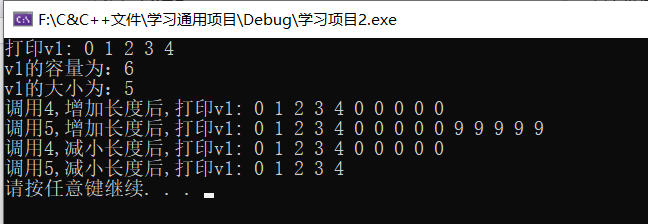4.vector的插入和删除

函数原型：
1.push_back(ele); //尾部插入元素ele
2.pop_back(); //删除最后一个元素
3.insert(const_iterator pos,ele); //在迭代器指向的位置pos处插入一个元素ele
4.insert(const_iterator pos,int count,ele); //在迭代器指向的位置pos处插入count个元素ele
5.erase(const_iterator pos); //删除迭代器指向的元素
6.erase(const_iterator begin,const_iterator end); //删除迭代器从begin到end之间的元素
7.clear(); //删除容器中所有元素

测试案例：

void text04()
{
vector<int> v1;
for (int i = 0; i < 5; ++i)
{
v1.push_back(6);//调用1，尾部插入元素6
}
cout << "打印v1: ";
printVector(v1);
v1.pop_back();//调用2，删除最后一个元素
cout << "调用2，删除最后一个元素后，打印v1: ";
printVector(v1);
v1.insert(v1.begin(),20);//调用3，在首位插入20
cout << "调用3，在首位插入20，打印v1: ";
printVector(v1);
v1.insert(v1.end(), 3, 20);//调用4，在尾部插入3个20
cout << "调用4，在尾部插入3个20，打印v1: ";
printVector(v1);
v1.erase(v1.begin()); //调用5，在首位删除一个元素
cout << "调用5，在首位删除一个元素，打印v1: ";
printVector(v1);
v1.erase(v1.begin(),v1.end()); //调用6，删除首位到末尾所有元素，也就是删除全部元素
cout << "调用6，删除首位到末尾所有元素，打印v1: ";
printVector(v1);
v1.clear();//调用7，清空所有元素
}

测试结果：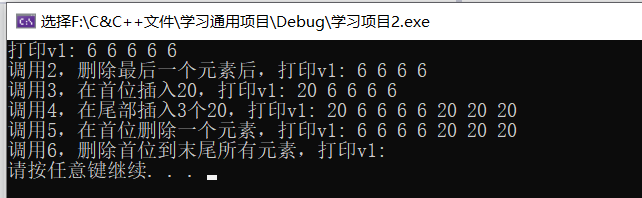5.vector数据存取

函数原型：
1.at(int idx); //返回索引idx所指的数据
2.operator[]; //返回[]内索引所指的数据
3.front(); //返回容器中第一个元素
4.back(); //返回容器中最后一个元素

测试案例：

void text05()
{
vector<int> v;
for (int i = 0; i < 5; ++i)
{
v.push_back(i);
}
//利用at访问v
cout << "调用1，打印v: ";
for (int i = 0; i < v.size(); ++i)
{
cout << v.at(i) << " ";//调用1
}
cout << endl;
//利用[]访问v
cout << "调用2，打印v: ";
for (int i = 0; i < v.size(); ++i)
{
cout << v[i] << " ";//调用2
}
cout << endl;
cout << "容器中第一个元素是：" << v.front() << endl;//调用3
cout << "容器中最后一个元素是：" << v.back() << endl;//调用4
}

测试结果：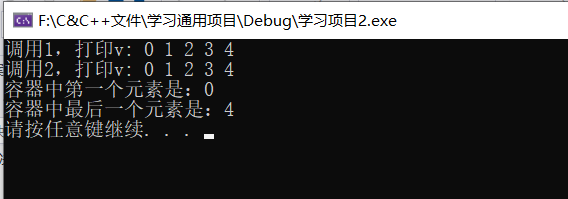6.vector互换容器

函数原型：
swap(v); //容器v和当前容器互换

测试案例1：基本使用

void text06_1()
{
vector<int> v1,v2;
for (int i = 0; i < 10; ++i)
{
v1.push_back(i);
v2.push_back(9 - i);
}
cout << "交换前：" << endl;
printVector(v1);
printVector(v2);
cout << "交换后：" << endl;
v1.swap(v2);   //调用互换函数
printVector(v1);
printVector(v2);
}

测试结果：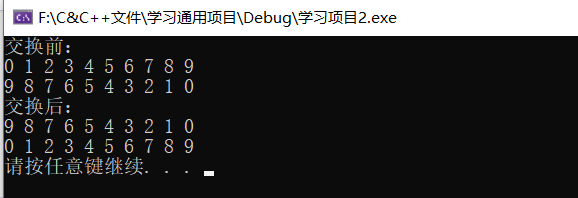测试案例2：实际用途

void text06_2()
{
vector<int> v;
for (int i = 0; i < 1000; ++i)
{
v.push_back(i);
}
cout << "初始时：" << endl;
cout << "v的容量：" << v.capacity() << endl;
cout << "v的大小：" << v.size() << endl;

cout << "重新指定空间后：" << endl;
v.resize(10);
cout << "v的容量：" << v.capacity() << endl;
cout << "v的大小：" << v.size() << endl;

//巧用swap收缩内存
cout << "swap收缩内存后：" << endl;
vector<int>(v).swap(v);   //vector<int>(v) 是创建一个匿名对象，并拷贝v的数据
//以此匿名对象与v交换，交换完后系统自动删除匿名对象
cout << "v的容量：" << v.capacity() << endl;
cout << "v的大小：" << v.size() << endl;
}

测试结果：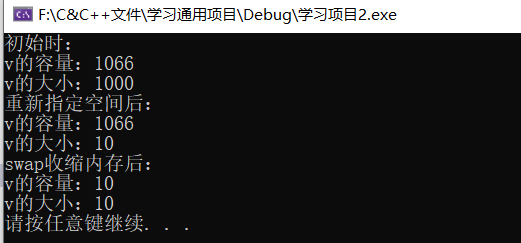7.vector预留空间

功能：减少vector在动态扩容时的扩展次数
每次使用push_back(v)时，如果容器v的大小要超过v的容量时，系统就会对v进行一次动态扩容，至于扩大多少空间，由系统决定

函数原型：
reserve(int len);//容器预留len个元素长度，也就是把容量扩为len，
预留的位置并不初始化，同时也不可访问

测试案例：

void text07()
{
vector<int> v1,v2;
v2.reserve(10000);//给v2设置预留空间,v1不设置

//对比v1,v2，系统需要动态扩容多少次
int num1 = 0;       //扩容次数
int num2 = 0;
int capacity1 = 0;  //容量大小
int capacity2 = 0;
for (int i = 0; i < 10000; ++i)
{
v1.push_back(i);
if (capacity1 != v1.capacity())//计算扩容次数,当容量不相等时，证明系统扩容了
{
capacity1 = v1.capacity();
++num1;
}

v2.push_back(i);
if (capacity2 != v2.capacity())
{
capacity2 = v2.capacity();
++num2;
}
}
cout << "系统对v1扩容" << num1 << "次" << endl;
cout << "系统对v2扩容" << num2 << "次" << endl;
}

测试结果：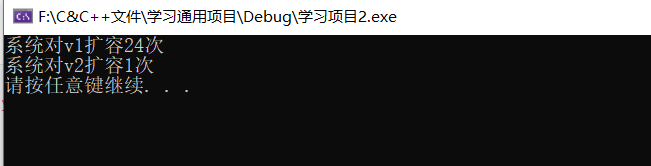写在最后

vector容器算是C++中最常用的容器之一了，我在真正学习它之前就经常用了，看多了别人怎么用，自己也就大概会一点了，但是那时候不知道它的具体原理，现在学习完了，详细总结了vector的具体用法供大家一起学习，喜欢的话，点赞收藏加关注喽！

展开全文容器 c++
• 向量(Vector)是一个封装了动态大小数组的顺序容器(Sequence Container)。跟任意其它类型容器一样，它能够存放各种类型的对象。可以简单的认为，向量是一个能够存放任意类型的动态数组。二、容器特性1.顺序序列顺序...

一、什么是vector？

向量(Vector)是一个封装了动态大小数组的顺序容器(Sequence Container)。跟任意其它类型容器一样，它能够存放各种类型的对象。可以简单的认为，向量是一个能够存放任意类型的动态数组。

二、容器特性

1.顺序序列

顺序容器中的元素按照严格的线性顺序排序。可以通过元素在序列中的位置访问对应的元素。

2.动态数组

支持对序列中的任意元素进行快速直接访问，甚至可以通过指针算述进行该操作。操供了在序列末尾相对快速地添加/删除元素的操作。

3.能够感知内存分配器的(Allocator-aware)

容器使用一个内存分配器对象来动态地处理它的存储需求。

三、基本函数实现

1.构造函数

vector():创建一个空vector

vector(int nSize):创建一个vector,元素个数为nSize

vector(int nSize,const t& t):创建一个vector，元素个数为nSize,且值均为t

vector(const vector&):复制构造函数

vector(begin,end):复制[begin,end)区间内另一个数组的元素到vector中

2.增加函数

void push_back(const T& x):向量尾部增加一个元素X

iterator insert(iterator it,const T& x):向量中迭代器指向元素前增加一个元素x

iterator insert(iterator it,int n,const T& x):向量中迭代器指向元素前增加n个相同的元素x

iterator insert(iterator it,const_iterator first,const_iterator last):向量中迭代器指向元素前插入另一个相同类型向量的[first,last)间的数据

3.删除函数

iterator erase(iterator it):删除向量中迭代器指向元素

iterator erase(iterator first,iterator last):删除向量中[first,last)中元素

void pop_back():删除向量中最后一个元素

void clear():清空向量中所有元素

4.遍历函数

reference at(int pos):返回pos位置元素的引用

reference front():返回首元素的引用

reference back():返回尾元素的引用

iterator begin():返回向量头指针，指向第一个元素

iterator end():返回向量尾指针，指向向量最后一个元素的下一个位置

reverse_iterator rbegin():反向迭代器，指向最后一个元素

reverse_iterator rend():反向迭代器，指向第一个元素之前的位置

5.判断函数

bool empty() const:判断向量是否为空，若为空，则向量中无元素

6.大小函数

int size() const:返回向量中元素的个数

int capacity() const:返回当前向量所能容纳的最大元素值

int max_size() const:返回最大可允许的vector元素数量值

7.其他函数

void swap(vector&):交换两个同类型向量的数据

void assign(int n,const T& x):设置向量中前n个元素的值为x

void assign(const_iterator first,const_iterator last):向量中[first,last)中元素设置成当前向量元素

8.看着清楚

1.push_back 在数组的最后添加一个数据

2.pop_back 去掉数组的最后一个数据

3.at 得到编号位置的数据

4.begin 得到数组头的指针

5.end 得到数组的最后一个单元+1的指针

6．front 得到数组头的引用

7.back 得到数组的最后一个单元的引用

8.max_size 得到vector最大可以是多大

9.capacity 当前vector分配的大小

10.size 当前使用数据的大小

11.resize 改变当前使用数据的大小，如果它比当前使用的大，者填充默认值

12.reserve 改变当前vecotr所分配空间的大小

13.erase 删除指针指向的数据项

14.clear 清空当前的vector

15.rbegin 将vector反转后的开始指针返回(其实就是原来的end-1)

16.rend 将vector反转构的结束指针返回(其实就是原来的begin-1)

17.empty 判断vector是否为空

18.swap 与另一个vector交换数据

四、基本用法

#include < vector>

using namespace std;

五、简单介绍

Vector标识符

Vector标识符(最大容量)

Vector标识符(最大容量,初始所有值)

Int i={1,2,3,4,5}

Vectorvi(I,i+2);//得到i索引值为3以后的值

Vector< vector< int> >v; 二维向量//这里最外的<>要有空格。否则在比较旧的编译器下无法通过

实例

1.pop_back()&push_back(elem)实例在容器最后移除和插入数据

实例

#include#include#includeusingnamespacestd;intmain(){vectorobj;//创建一个向量存储容器 intfor(inti=0;i<10;i++)// push_back(elem)在数组最后添加数据{obj.push_back(i);cout<

输出结果为：

0,1,2,3,4,5,6,7,8,9,

0,1,2,3,4,

2.clear()清除容器中所有数据

实例

#include#include#includeusingnamespacestd;intmain(){vectorobj;for(inti=0;i<10;i++)//push_back(elem)在数组最后添加数据{obj.push_back(i);cout<

输出结果为：

0,1,2,3,4,5,6,7,8,9,

3.排序

实例

#include#include#include#includeusingnamespacestd;intmain(){vectorobj;obj.push_back(1);obj.push_back(3);obj.push_back(0);sort(obj.begin(),obj.end());//从小到大cout<

输出结果为：

从小到大:

0,1,3,

从大到小:

3,1,0,

1.注意 sort 需要头文件 #include

2.如果想 sort 来降序，可重写 sort

bool compare(int a,int b)

{

return a< b; //升序排列，如果改为return a>b，则为降序

}

int a={2,4,1,23,5,76,0,43,24,65},i;

for(i=0;i<20;i++)

cout<< a[i]<< endl;

sort(a,a+20,compare);

4.访问(直接数组访问&迭代器访问)

实例

#include#include#include#includeusingnamespacestd;intmain(){//顺序访问vectorobj;for(inti=0;i<10;i++){obj.push_back(i);}cout<::iteratorit;//声明一个迭代器，来访问vector容器，作用：遍历或者指向vector容器的元素for(it=obj.begin();it!=obj.end();it++){cout<

输出结果为：

直接利用数组：0 1 2 3 4 5 6 7 8 9

利用迭代器：0 1 2 3 4 5 6 7 8 9

5.二维数组两种定义方法(结果一样)

方法一

#include#include#include#includeusingnamespacestd;intmain(){intN=5,M=6;vector >obj(N);//定义二维动态数组大小5行for(inti=0;i

方法二

#include#include#include#includeusingnamespacestd;intmain(){intN=5,M=6;vector >obj(N,vector(M));//定义二维动态数组5行6列for(inti=0;i

输出结果为：

0 0 0 0 0 0

0 0 0 0 0 0

0 0 0 0 0 0

0 0 0 0 0 0

0 0 0 0 0 0

展开全文• } 5 vector插入【push_back()、insert()】和删除【pop_back()、erase()、clear()】 （1）vector容器插入元素：使用成员函数push_back(..)、insert(..)向vector容器插入元素。 函数原型： ①push_back(ele);：尾插法...

1 vector基本概念

作用单端数组，仅可在容器的尾部插入或删除元素，与数组非常相似。
区别普通数组静态空间vector支持动态扩展容量机制。

动态扩展：并非在原有内存空间中扩展新空间，而是申请更大的内存空间，将原有数据拷贝至新的内存空间，并释放原有内存空间

vector容器的迭代器：支持随机访问的迭代器，可跳跃式访问容器元素。push_back()尾插法插入数据。
pop_back()尾删法删除数据。
front()：获取vector容器的第1个元素
back()：获取vector容器的最后1个元素
begin()：获取起始迭代器，指向容器中的第1个元素
end()：获取结束迭代器，指向容器中的最后1个元素下一个位置

2 vector构造函数

作用：创建vector容器。

注：使用vector容器时，需包含头文件#include <vector>

函数原型
（1）vector<T> v;：默认无参构造函数，采用类模板实现。
（2）vector(v.begin(), v.end());：拷贝容器对象v[begin(), end())区间左闭右开，包左不包右）的元素进行初始化。
（3）vector(n, elem);：有参构造函数，使用nelem元素进行初始化。
（4）vector(const vector &vec);：拷贝构造函数，使用已有vector对象初始化新的对象。

注：通常使用默认无参构造函数拷贝构造函数实例化vector容器对象。

示例：vector构造函数

#include <iostream>
using namespace std;
#include <vector>	//包含头文件

//打印vector元素的函数模板
template<typename T>
void printVector(vector<T>& v) {
//for循环遍历
for (vector<T>::iterator it = v.begin(); it != v.end(); it++) {
cout << (*it) << " ";
}
cout << endl;
}

int main() {
/* 1.默认无参构造函数 */
vector<int> v1;

//尾插法插入元素
for (int i = 0; i < 5; i++) {
v1.push_back(i);
}
//遍历
printVector<int>(v1);	//0 1 2 3 4

/* 2.有参构造，区间拷贝 */
//左闭右开区间的元素
vector<int> v2(v1.begin(), v1.end());
printVector<int>(v2);	//0 1 2 3 4

/* 3.n个elem元素赋值 */
//5个整型元素6
vector<int> v3(5, 6);
printVector<int>(v3);	//6 6 6 6 6

/* 4.拷贝构造函数 */
vector<int> v4(v3);
printVector<int>(v4);	//6 6 6 6 6

return 0;
}

3 vector赋值操作【operator=、assign()】

作用：通过重载赋值运算符operator=成员函数assign()，对vector容器进行赋值。

函数原型
（1）vector& operator=(const vector &vec);：重载赋值运算符，使用目标vector容器，对当前vector容器赋值。
（2）assign(begin, end);：拷贝目标vector容器中[begin(), end())区间左闭右开，包左不包右）的元素，对当前vector容器赋值。
（3）assign(n, elem);：使用nelem元素，对当前vector容器赋值。

示例：vector赋值操作

#include <iostream>
using namespace std;
#include <vector>	//包含头文件

//打印vector元素的函数模板
template<typename T>
void printVector(vector<T>& v) {
//for循环遍历
for (vector<T>::iterator it = v.begin(); it != v.end(); it++) {
cout << (*it) << " ";
}
cout << endl;
}

int main() {
vector<int> v;

//尾插法插入元素
for (int i = 0; i < 5; i++) {
v.push_back(i);
}
//遍历
printVector<int>(v);	//0 1 2 3 4

/* 1.重载运算符=赋值 */
vector<int> v1 = v;
printVector<int>(v1);	//0 1 2 3 4

/* 2.assign()函数，区间拷贝 */
//左闭右开区间的元素
vector<int> v2;
v2.assign(v.begin(), v.end());
printVector<int>(v2);	//0 1 2 3 4

/* 3.assign()函数，n个elem元素赋值 */
vector<int> v3;
//6个整型元素6
v3.assign(6, 6);
printVector<int>(v3);	//6 6 6 6 6 6

return 0;
}

4 vector容量【capacity()】和大小【size()、resize()】

作用：操作vector容器的容量大小（即元素个数）。

注：容器的容量始终大于等于容器的大小（即元素个数），并支持动态扩展容量

函数原型
（1）empty();：判断容器是否为空。
（2）capacity();：获取容器的容量
（3）size();：获取容器的大小，即元素个数
（4）resize(int num);重新指定容器的长度为num
若容器变长，则以默认值0填充新位置；若容器变短，则容器末尾超出新长度的元素被删除
（5）resize(int num, elem);重新指定容器的长度为num
若容器变长，则以指定值elem填充新位置；若容器变短，则容器末尾超出新长度的元素被删除

示例：vector容量和大小

#include <iostream>
using namespace std;
#include <vector>	//包含头文件

//打印vector元素的函数模板
template<typename T>
void printVector(vector<T>& v) {
//for循环遍历
for (vector<T>::iterator it = v.begin(); it != v.end(); it++) {
cout << (*it) << " ";
}
cout << endl;
}

int main() {
vector<int> v1;
//尾插法插入元素
for (int i = 0; i < 5; i++) {
v1.push_back(i);
}
printVector<int>(v1);	//0 1 2 3 4

//empty()：判断容器是否为空
cout << (v1.empty() ? "v1为空" : "v1不为空") << endl;	//v1不为空

//capacity(); ：获取容器的容量。
cout << "v1的容量：" << v1.capacity() << endl;		//6（随机值）

//size(); ：获取容器的大小，即元素个数。
cout << "v1的大小/元素个数：" << v1.size() << endl;	//5

//resize(int num);：重新指定容器的长度为num
//若容器变长，则以默认值0填充新位置；若容器变短，则容器末尾超出新长度的元素被删除。
v1.resize(10);			//长度变大时，使用默认值0填充
printVector<int>(v1);	//0 1 2 3 4 0 0 0 0 0

v1.resize(3);			//长度变小时，容器末尾超出新长度的元素被删除
printVector<int>(v1);	//0 1 2

//resize(int num, elem); ：重新指定容器的长度为num。
//若容器变长，则以指定值elem填充新位置；若容器变短，则容器末尾超出新长度的元素被删除。
v1.resize(8, 6);		//长度变大时，使用指定值6填充
printVector<int>(v1);	//0 1 2 6 6 6 6 6

return 0;
}

5 vector插入【push_back()、insert()】和删除【pop_back()、erase()、clear()】

（1）vector容器插入元素：使用成员函数push_back(..)insert(..)向vector容器插入元素。

函数原型
push_back(ele);尾插法，向vector容器的尾部插入元素ele
insert(const_iterator pos, ele);迭代器指向位置pos插入元素ele
insert(const_iterator pos, int n, ele);迭代器指向位置pos插入n个元素ele

（2）vector容器删除元素：使用成员函数pop_back(..)erase(..)clear(..)删除vector容器中的元素。

函数原型
pop_back();尾删法，删除vector容器尾部的最后1个元素
erase(const_iterator pos);：删除迭代器指向位置的元素。
erase(const_iterator start, const_iterator end);：删除迭代器指向位置[start, end)区间的所有元素。
clear();：清空容器中的所有元素

注1：清空容器的所有元素，v.clear();等价于v.erase(v.begin(), v.end());
注2：插入元素insert(..)、删除元素erase(..)均需要迭代器对象作为参数。

示例：vector插入和删除元素

#include <iostream>
using namespace std;
#include <vector>	//包含头文件

//打印vector元素的函数模板
template<typename T>
void printVector(vector<T>& v) {
//for循环遍历
for (vector<T>::iterator it = v.begin(); it != v.end(); it++) {
cout << (*it) << " ";
}
cout << endl;
}

int main() {
vector<int> v;

//插入元素：push_back()
v.push_back(1);
v.push_back(2);
v.push_back(3);
v.push_back(4);
v.push_back(5);
printVector<int>(v);	//1 2 3 4 5

//删除元素：pop_back()
v.pop_back();
printVector<int>(v);	//1 2 3 4

//插入元素：insert(const_iterator pos, ele); 迭代器指向位置pos插入元素ele
//在起始迭代器的指向位置插入元素10
v.insert(v.begin(), 10);
printVector<int>(v);	//10 1 2 3 4

//插入元素：insert(const_iterator pos, int n, ele); 迭代器指向位置pos插入n个元素ele。
//在起始迭代器的指向位置插入2个元素0
v.insert(v.begin(), 2 , 0);
printVector<int>(v);	//0 0 10 1 2 3 4

//删除元素：erase(const_iterator pos); 删除迭代器指向位置的元素
//删除起始迭代器的指向位置的元素
v.erase(v.begin());
printVector<int>(v);	//0 10 1 2 3 4

//删除元素：erase(const_iterator start, const_iterator end); 删除迭代器指向位置[start, end)区间的所有元素。
//删除起始迭代器后一个位置 至 结束迭代器 之间区间的所有元素
v.erase(++v.begin(), v.end());
printVector<int>(v);	//0

//清空元素：clear(); 清空容器中的所有元素。
v.clear();
printVector<int>(v);	//（空）

return 0;
}

6 vector数据存取【operator[]、at()】

作用：通过重载赋值运算符operator[]成员函数at(int index)，对vector容器的单个元素进行（作为右值）或（作为左值）。

函数原型
（1）operator[](int index);：重载运算符[]，读写指定索引位置index的元素。
（2）at(int index);：成员函数，读写指定索引位置index的元素。
（3）front();：返回容器的第1个元素
（4）back();：返回容器的最后1个元素

访问/遍历vector容器元素的方式
迭代器
重载运算符[]
成员函数at()

示例：vector读写元素

#include <iostream>
using namespace std;
#include <vector>	//包含头文件

//打印vector元素的函数模板
template<typename T>
void printVector(vector<T>& v) {
//for循环遍历
for (vector<T>::iterator it = v.begin(); it != v.end(); it++) {
cout << (*it) << " ";
}
cout << endl;
}

int main() {
vector<int> v;
//尾插法插入元素
for (int i = 0; i < 5; i++) {
v.push_back(i);
}

//遍历方式1：重载运算符
for (int i = 0; i < v.size(); i++) {
//重载运算符[]：读写指定索引位置index的元素。
cout << v[i] << " ";		//0 1 2 3 4
}
cout << endl;

//遍历方式2：成员函数at()
for (int i = 0; i < v.size(); i++) {
//重载运算符[]：读写指定索引位置index的元素。
cout << v.at(i) << " ";		//0 1 2 3 4
}
cout << endl;

//修改元素1：重载运算符
v *= 10;
printVector<int>(v);	//0 1 2 3 40

//修改元素2：成员函数at()
v.at(0) = -1;
printVector<int>(v);	//-1 1 2 3 40

//获取第1个元素
cout << "第1个元素：" << v.front() << endl;	//-1

//获取最后1个元素
cout << "最后1个元素：" << v.back() << endl;	//40

return 0;
}

7 vector互换容器【swap()】

函数原型swap(vec);：将目标vector容器vec的元素与自身互换。

作用
（1）实现两个vector容器的元素互换

（2）可结合匿名对象拷贝构造函数，实现容器内存收缩，如：vector<int>(v).swap(v);
背景：当容量较大的vector容器，使用成员函数resize()将容器的大小（元素个数）调整至较小值时，vector容器实际容量不变，导致内存资源浪费
内存收缩步骤
vector<int>(v)：调用拷贝构造函数创建vector类的匿名对象，将根据原vector容器对象v的大小（元素个数）分配一定的容量（较小值）。
vector<int>(v).swap(v);：原vector对象v和匿名对象实现容器互换，即指针指向的内存地址互换，匿名对象指向具有较大容量的内存空间。
匿名对象调用完毕后立即释放，对应的较大容量的内存空间被自动释放。
④原vector对象v现指向具有较小容量的内存空间，实现容器内存收缩示例：vector容器互换

#include <iostream>
using namespace std;
#include <vector>	//包含头文件

//打印vector元素的函数模板
template<typename T>
void printVector(vector<T>& v) {
//for循环遍历
for (vector<T>::iterator it = v.begin(); it != v.end(); it++) {
cout << (*it) << " ";
}
cout << endl;
}

//vector容器互换
void func1() {
vector<int> v1;
for (int i = 0; i < 5; i++) {
v1.push_back(i);
}

vector<int> v2;
for (int i = 4; i >= 0; i--) {
v2.push_back(i);
}

cout << "交换前v1容器：" << endl;
printVector<int>(v1);	//0 1 2 3 4
cout << "交换前v2容器：" << endl;
printVector<int>(v2);	//4 3 2 1 0

//容器互换
v1.swap(v2);

cout << "交换后v1容器：" << endl;
printVector<int>(v1);	//4 3 2 1 0
cout << "交换后v2容器：" << endl;
printVector<int>(v2);	//0 1 2 3 4
}

//实际作用：vector容器内存收缩
void func2(){
//现有容器v开始时具有较大容量和较多元素
vector<int> v;
for (int i = 0; i < 100000; i++) {
v.push_back(i);
}
cout << "resize前..." << endl;
cout << "v的容量:" << v.capacity() << endl;	//容量：138255
cout << "v的大小:" << v.size() << endl;		//大小：100000

/* resize() 重新指定大小，长度为5 */
v.resize(5);

//resize容器大小后，容量保持【较大值】不变，容器大小变为【较小值】
//内存资源浪费
cout << "resize后..." << endl;
cout << "v的容量:" << v.capacity() << endl;	//容量：138255
cout << "v的大小:" << v.size() << endl;		//大小：5

/* 创建匿名对象，并进行容器互换 */
vector<int>(v).swap(v);

//容器互换后，原vector容器的容量和大小均变为【较小值】，实现容器内存收缩
//匿名对象调用完毕后立即释放，指向的较大内存空间被释放
cout << "容器互换后..." << endl;
cout << "v的容量:" << v.capacity() << endl;	//容量：5
cout << "v的大小:" << v.size() << endl;		//大小：5
}

int main() {
//func1();
func2();

return 0;
}

8 vector预留空间【reserve()】

作用：当vector容器存储的数据量较大时，一开始可利用成员函数reserve()预留指定大小的内存空间，减少vector容器在动态扩展容量时的扩展次数，降低数据拷贝过程的资源消耗。

函数原型
reserve(int len);：容器预留len个元素长度的内存空间，但预留内存空间不进行初始化不可访问

注：reserve(...)reverse()需注意区分。
reserve(...)用于vector容器预留空间
reverse(...)用于反转容器的元素，如reverse(v.begin(), v.end());

示例：vector预留空间

#include <iostream>
using namespace std;
#include <vector>	//包含头文件

//vector预留空间
void func() {
vector<int> v;

/* 预留空间 */
//v.reserve(10000);		//动态扩展7次
//v.reserve(20000);		//动态扩展5次
//v.reserve(50000);		//动态扩展3次
v.reserve(100000);		//动态扩展1次

//统计vector插入100000个元素时，动态扩展容量的总次数
int count = 0;
//初始化空指针，用于判断是否动态扩展
int* p = NULL;

for (int i = 0; i < 100000; i++) {
v.push_back(i);

//判断指向vector容器首元素的指针，记录的地址值是否发生改变
//首地址发生改变 → vector容器已动态扩展
if (p != &v) {
//更新指针的地址值
p = &v;
count++;
//cout << "vector容器的首地址：" << p << endl;
}
}
cout << "动态扩展容量的总次数：" << count << endl;
//未预留空间时：动态扩展30次
//预留10000个元素长度时：动态扩展7次
//预留20000个元素长度时：动态扩展5次
//预留50000个元素长度时：动态扩展3次
//预留100000个元素长度时：动态扩展1次
}

int main() {
func();

return 0;
}

展开全文c++ stl 容器 vector
• 本题同PAT1025 但1025未使用vector容器 错误原因: vector<struct Node> v(n); for (int i = add; i != -1; i = Node[i].next) { v[a++] = Node[i]; //v.push_back(Node[i]); //这种.stl c++
• vector容器是一个动态数组的结构，在内存中有一个指针指向一块连续的内存。类似数组结构一样。它的特点支持随机访问数据，因为其在内存中的单元是连续。它可以容纳许多类型的数据，如若干个整数，所以称其为容器。...c++
• vector 容器是数组的最佳替代者 vector 容器同样可以用于保存和管理大量同类型数据，但无论是在内存管理上还是在对数据元素的访问上，都要优于数组： vector 容器动态增减的容量大小替代了数组的固定容量，可以适应...c++ stl
• map容器与vector容器的混用 int number; while (query.next()) { for (int i = 0; i < 36; i++) { number = i; m[j] = query.value(i).toString(); a.insert(number, m); } }### 三级标题 四级...stl
• 1、功能： vector数据结构和数组非常相似，也称为单端数组 ...4、vector容器的两种赋值操作（构造函数和 =） #include<iostream> #include<string> #include"myarray.hpp" #include<vector> #c++ 数据结构
• vector<int> v; v=1;//错误，因为v不包含任何元素 ...根据《c++ primer》page.93中提到，不能下标形式添加元素，但是可以对下标形式对元素内容进行修改，即下标v[n]是返回v中第n个位置元素的引用。 ...c++
• 1.对vector的容量和大小进行操作。 2.函数原型： empty(); //判断容器是否为空,返回值为真则代表容器空 capacity(); //容器的容量 size(); //返回容器中元素的个数 resize(int num); //重新制定容器的长度为num...
• vector容器的两种insert方法： 1: insert(const_iterator pos, int count,element);//迭代器指向位置pos插入count个元素element #if __cplusplus >= 201103L /** * @brief Inserts a number of copies of given...数据结构 stl
• 1.用法就是大白话，对vector容器插入和删除。 2.函数原型： push_back(ele); //尾部插入元素ele pop_back(); //删除最后一个元素 insert(const_iterator pos, ele); //迭代器指向位置pos插入元素ele insert...
• 1获得容器最后一个元素------使用back或rbegin取得//back、rbegin有常量和引用两种形式std::vectormyVector;myVector.back()=3;std::vector::reverse_iteratortailIter;tailIter=myVector.rbegin();*tailIter=32删除...
• vector排序 sort(arr.begin() , arr.end()) 创建vector带有初始值 vector<int> arr (len , -1); 创建一个以len为长度的，内容全为-1的vectorc++ vector 数据结构
• 基础不牢,在容器vector上撞墙,做做笔记来吸取教训 vector添加元素,我原来的代码 vector<MetricPoint2D> Smooth::smoothPath(const vector<MetricPoint2D> &path) { int length = path.size(); ...c++
• vector容器

2021-07-06 15:54:10
使用vector需要包含头文件 什么是vector 向量是一个能够存放任意类型的动态数组。 初始化 一、不带参数 // 定义了一个int类型的容器 vector<int> v1; // 定义了一个double类型的容器 vector<double> v2;...c++
• # include<iostream> using namespace std;...//容器构造 void test01() { //无参构造 vector<int>v1; for (int i = 0; i < 10; i++) { v1.push_back(i); } printVector(v1); vector<.容器 c++ 运维
• #include<iostream>//c++中标准的输入和输出流... v)//打印输出vector容器的所有元素 {//for循环第一个参数是找到起始迭代器的位置 // //第二个参数是 for循环退出的条件， // //d.end()指向的是终止迭代器的c++ vector容器
• 关于STL中vector容器的学习，编译运行后边看代码，边看执行结果效果更佳，还是想说看别人的代码一百遍，不如自己动手写一遍。vector向量容器不但能像数组一样对元素进行随机访问，还能随时在尾部插入元素，简单而...
• vector容器详解

2021-10-19 16:30:28
vector数据结构和数组非常相似，也称为单端数组。vector可以说是一个动态数组，它可以存储任何类型的数据，包括...vector容器的迭代器是支持随机访问的迭代器 使用vector需包含头文件#include< vector > 1. ...数据结构 c++
• STL vector容器

2021-04-01 23:50:34
vector容器的常用接口及用法： vector：STL 中最常用的容器，和数组结构非常类似，也被称作单端数组； vector 容器和普通数组的区别：它们的不同之处在于，数组是静态空间（一旦给定了初始长度，无法修改），而 ...c++ stl 数组
• vector容器中数据的排序方法

千次阅读 2021-12-13 16:51:53
在项目中经常会遇到对vector容器中数据排序的情况，有时候vector中数据还常常是pair关联容器。此处给出一个使用demo,使用了C++中的lambda表达式。 #include"algorithm" #include"vector" using namespace std; ...容器 c++ 运维
•容器 c++ stl
• vector的clear()操作不能用于空容器 例如 #include<bits/stdc++.h> using namespace std; int main(){ int n; cin>>n; vector<int>f(n); f.clear(); f=1; } 这样就会报错（容器下标越界） 应...c++
• #include<...//vector容器嵌套容器 void test1() { vector<vector<int>>v; //创建小容器 vector<int>v1; vector<int>v2; vector<int>v3; //向小容器中添加数.容器 c++ 运维
• 使用vector容器的头文件是#include< vector > vector概念： vector容器常被成为向量容器(据说是线性代数中的一维数组就是叫做向量) vector是一个动态大小数组的顺序容器,可以认为vector是存放任意类型的动态...c++
• vector容器进行赋值 函数原型： 代码如下： #include <iostream> using namespace std; #include <vector> //vector赋值操作 void printVector(vector<int> &v) { for (vector<int &...c++ 数据结构
• deque是一个双端数组的好处就是，在头部的插入和删除很容易，因为头部有开口，但是对于vector容器而言，因为他是一个单端数组，所以它在进行头插的时候，必须要将所有的元素都往后面挪一位，当数据量大的时候，效率...c++...